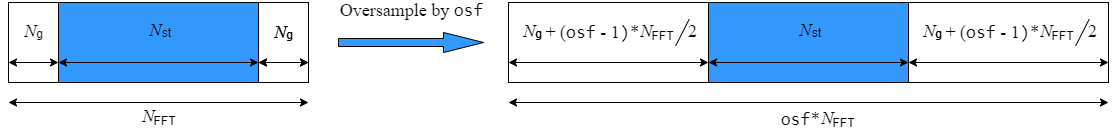wlanHTData

Generate HT-Data field waveform

Description

example

y = wlanHTData(psdu,cfg) generates an HT-Data field time-domain waveform for PLCP service data unit psdu and specified transmission parameters cfg. See HT-Data Field Processing for waveform generation details.

y = wlanHTData(psdu,cfg,scramInit) uses scramInit for the scrambler initialization state.

y = wlanHTData(___,OversamplingFactor=osf) generates an HT-Data field waveform with an oversampling factor. For more information about oversampling, see FFT-Based Oversampling.

Examples

collapse all

Generate the waveform signal for a 40 MHz HT-mixed data field with multiple transmit antennas. Create an HT format configuration object. Specify 40 MHz channel bandwidth, two transmit antennas, and two space-time streams.

cfgHT = wlanHTConfig('ChannelBandwidth','CBW40','NumTransmitAntennas',2,'NumSpaceTimeStreams', 2,'MCS',12)
cfgHT =
wlanHTConfig with properties:

ChannelBandwidth: 'CBW40'
NumTransmitAntennas: 2
NumSpaceTimeStreams: 2
SpatialMapping: 'Direct'
MCS: 12
GuardInterval: 'Long'
ChannelCoding: 'BCC'
PSDULength: 1024
AggregatedMPDU: 0
RecommendSmoothing: 1

Assign PSDULength bytes of random data to a bit stream and generate the HT data waveform.

PSDU =  randi([0 1],cfgHT.PSDULength*8,1);
y = wlanHTData(PSDU,cfgHT);

Determine the size of the waveform.

size(y)
ans = 1×2

2080           2

The function returns a complex two-column time-domain waveform. Each column contains 2080 samples, corresponding to the HT-Data field for each transmit antenna.

Input Arguments

collapse all

PLCP Service Data Unit (PSDU), specified as an Nb-by-1 vector. Nb is the number of bits and equals PSDULength × 8.

Data Types: double

Transmission parameters, specified as a wlanHTConfig object.

Scrambler initialization state for each packet generated, specified as an integer in the interval [1, 127] or as the corresponding binary vector of length seven. The default value of 93 is the example state given in IEEE Std 802.11™-2012, Section L.1.5.2.

The scrambler initialization used on the transmission data follows the process described in IEEE® Std 802.11-2012, Section 18.3.5.5 and IEEE Std 802.11ad™-2012, Section 21.3.9. The header and data fields that follow the scrambler initialization field (including data padding bits) are scrambled by XORing each bit with a length-127 periodic sequence generated by the polynomial S(x) = x7+x4+1. The octets of the PSDU are placed into a bit stream and, within each octet, bit 0 (LSB) is first and bit 7 (MSB) is last. This figure shows the generation of the sequence and the XOR operation.Conversion from integer to bits uses left-MSB orientation. For example, initializing the scrambler with decimal 1, the bits map to these elements.

ElementX7X6X5X4X3X2X1
Bit Value0000001

To generate the bit stream equivalent to a decimal, use the int2bit function. For example, for decimal 1:

int2bit(1,7)'
ans =

0     0     0     0     0     0     1

Example: [1; 0; 1; 1; 1; 0; 1] conveys the scrambler initialization state of 93 as a binary vector.

Data Types: double | int8

Oversampling factor, specified as a scalar greater than or equal to 1. The oversampled cyclic prefix length must be an integer number of samples.

Data Types: single | double | int8 | int16 | int32 | int64 | uint8 | uint16 | uint32 | uint64

Output Arguments

collapse all

HT-Data field time-domain waveform for HT-mixed format, returned as an NS-by-NT matrix. NS is the number of time domain samples, and NT is the number of transmit antennas.

collapse all

HT-Data field

The HT-Data field follows the last HT-long training field (HT-LTF) of an HT-mixed packet.The HT-Data field is carries one or more frames from the medium access control (MAC) layer and consists of four subfields.• Service — Contains 16 zeros to initialize the data scrambler

• PSDU — Variable-length field containing a PLCP service data unit (PSDU)

• Tail — Contains six zeros for each encoding stream, required to terminate a convolutional code

• Pad Bits — Variable-length field required to ensure that the HT-Data field consists of an integer number of symbols

PSDU

Physical layer (PHY) service data unit (PSDU). This field is composed of a variable number of octets. The minimum is 0 (zero) and the maximum is 2500. For more information, see IEEE Std 802.11™-2012, Section 15.3.5.7.

Algorithms

collapse all

HT-Data Field Processing

The HT-Data field follows the last HT-LTF in the packet structure.The HT-Data field includes the user payload in the PSDU, plus 16 service bits, 6 × NES tail bits, and additional padding bits as required to fill out the last OFDM symbol.

For algorithm details, refer to IEEE Std 802.11™-2012 , Section 20.3.11. The wlanHTData function performs transmitter processing on the HT-Data field and outputs the time-domain waveform for NT transmit antennas.NES is the number of BCC encoders. NSS is the number of spatial streams. NSTS is the number of space-time streams. NT is the number of transmit antennas.

BCC channel coding is shown. STBC and spatial mapping are optional modes for HT format.

FFT-Based Oversampling

An oversampled signal is a signal sampled at a frequency that is higher than the Nyquist rate. WLAN signals maximize occupied bandwidth by using small guardbands, which can pose problems for anti-imaging and anti-aliasing filters. Oversampling increases guardband width relative to the total signal bandwidth, thereby increasing the number of samples in the signal.

This function performs oversampling by using a larger IFFT and zero pad when generating an OFDM waveform. This diagram shows the oversampling process for an OFDM waveform with NFFT subcarriers comprising Ng guardband subcarriers on either side of Nst occupied bandwidth subcarriers.IEEE Std 802.11™-2012 IEEE Standard for Information technology — Telecommunications and information exchange between systems — Local and metropolitan area networks — Specific requirements — Part 11: Wireless LAN Medium Access Control (MAC) and Physical Layer (PHY) Specifications.

WLAN Toolbox Documentation평가판 신청